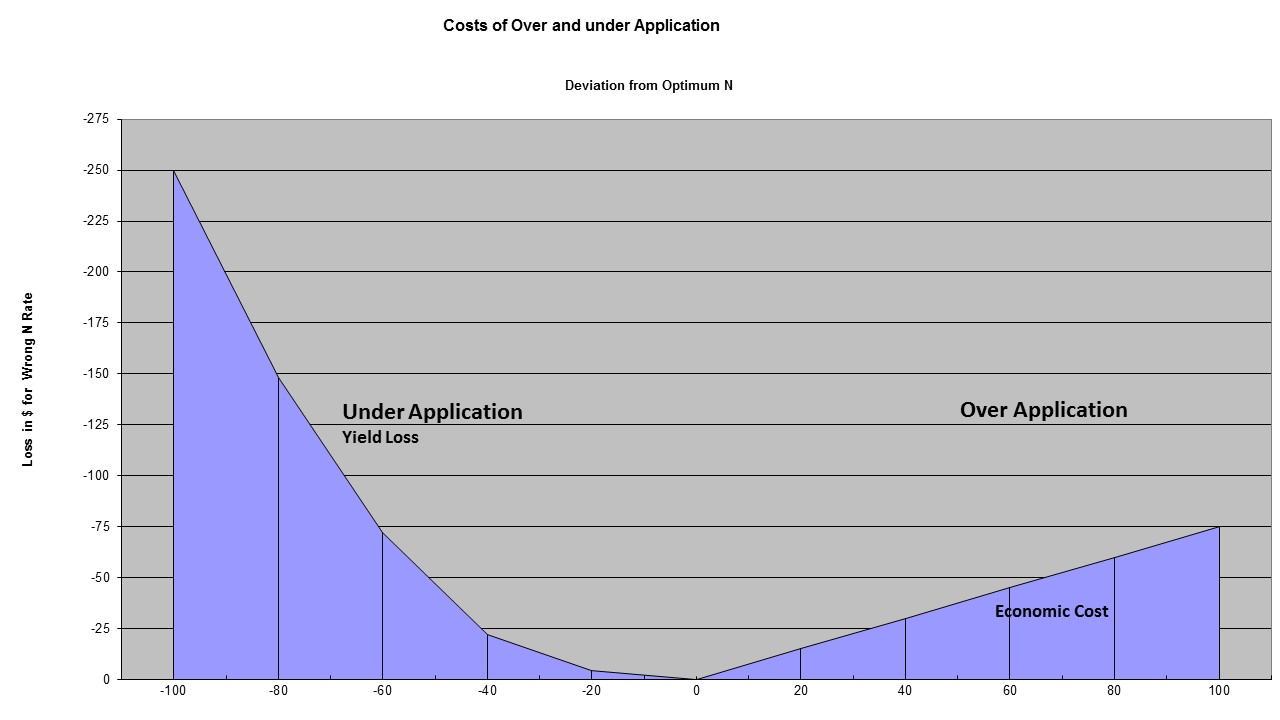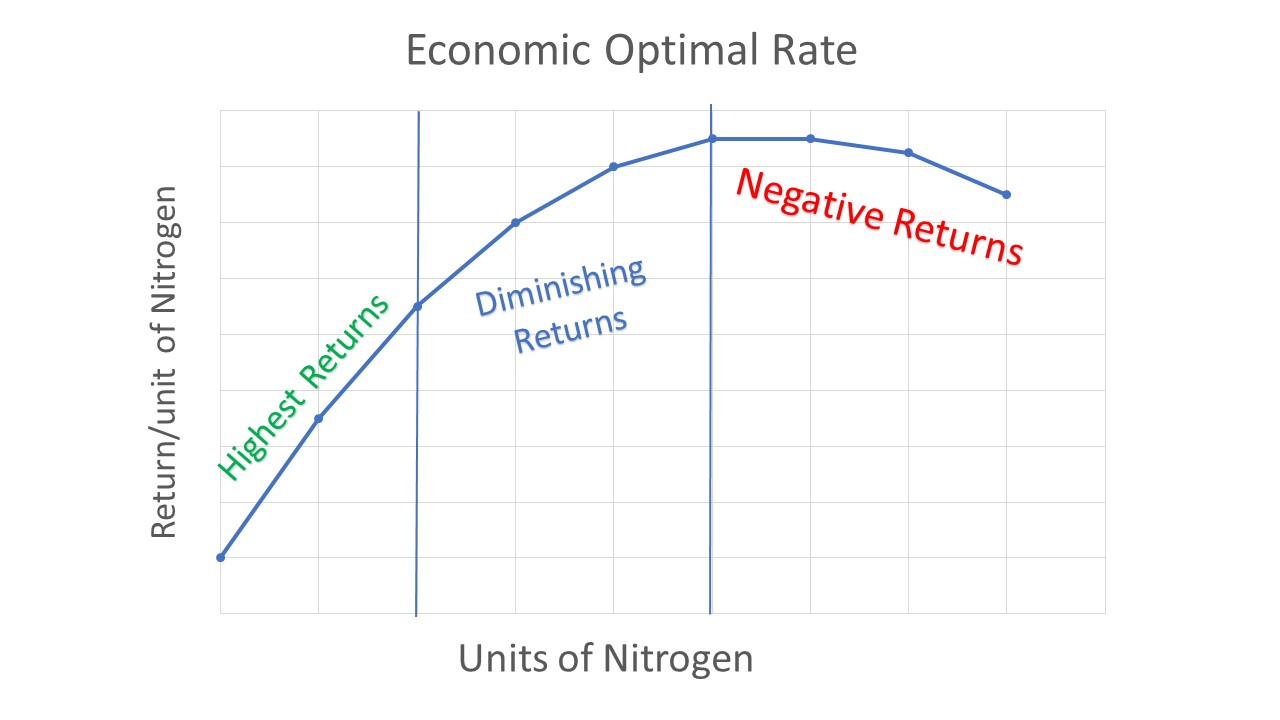## Delta Yield Concept

The delta yield concept simply looks at the change in yield as you apply nitrogen fertilizer. Implicit in this concept is that the soil provides a certain amount of nitrogen and as you apply fertilizer N you will get different levels of yield increase above what the soil provides for the crop. The response however, is not linear and and every pound of nitrogen fertilizer added will give less of a response than the previous pound.

Here at Cropsmith we believe an "active" approach to nitrogen sampling is best for managing nitrogen. With the Illinois Soil Nitrogen Test (ISNT) results and Pre-Sidedress Nitrate Test (PSNT) results, we can take the guesswork out of nitrogen applications and limit the over or under applications of nitrogen for growers.## Risk of Application

There is always a risk when applying fertilizer. If nitrogen is under applied, there will be a yield loss and farmers can miss out on maximum profits. Conversely, over application may not cover fertilizer cost, and contribute to nutrient contamination of water resources.

Applications within +/- 40 lbs of the Economic Optimum Nitrogen Rate (EONR) will likely limit the financial risk for growers. Using the innovative ISNT to better predict the soil mineralization of nitrogen, Cropsmith can give growers recommendations on nitrogen rates for every acre of their operation.## Economic Optimum Nitrogen Rate

Calculating delta yield for an area of a field requires a simple rate study. Rates in the study should include zero, your current nitrogen rate and rates that are higher and lower than your current rate by at least 40 lbs of N. Using the results to create a nitrogen response curve, one can calculate the most economical N rate. This is where the investment of additional nitrogen no longer increases yield enough to pay for the cost of fertilizer.

For each soil that would have a different response to N you would need to repeat the response study to determine the optimum N rate for the soil. Using the information generated by this type of study, Cropsmith can help you develop a variable rate nitrogen plan for your operation and improve how economically you can apply nitrogen.